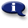# .

## Syllabus Information

Fall 2022
Oct 07,2022Use this page to maintain syllabus information, learning objectives, required materials, and technical requirements for the course.

Syllabus Information
MTH 052 - Math for Health and Physical Sciences
Associated Term: Fall 2022
Learning Objectives: Upon successful completion of this course, the student will be able to:
1. Practice decimal and fraction operations and apply to solve applications
2. Demonstrate the correct use of English, metric and apothecary units of measurement
3. Solve problems using unit (dimensional) analysis
4. Apply concepts of approximate numbers for rounding measurements
5. Demonstrate the correct use of labels for measurements, applications, and graphs
6. Use basic geometric formulas for area and perimeter of quadrilaterals
7. Compute with signed numbers
8. Solve equations and formulas for one variable
9. Interpret and create line graphs
10. Calculate and label slope of line graphs
11. Solve problems involving percents
12. Solve problems involving proportions for solutions and variation
13. Use and compare Fahrenheit, Celsius, and Kelvin temperature scales
14. Find the volume and density of objects
15. Calculate pH quantities involving logarithms
16. Calculate dosages for oral meds and injected meds including reconstituted liquids
17. Use and compute with exponential and scientific notation
18. Calculate both by hand and scientific calculator when appropriate
Required Materials:
Technical Requirements: